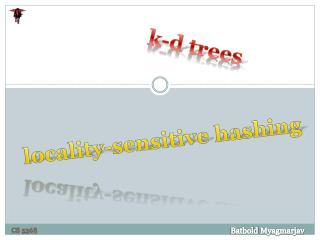DownloadDownload Presentationk -d trees

# k -d trees

Télécharger la présentation## k -d trees

- - - - - - - - - - - - - - - - - - - - - - - - - - - E N D - - - - - - - - - - - - - - - - - - - - - - - - - - -
##### Presentation Transcript

1. k-d trees locality-sensitive hashing BatboldMyagmarjav Cs 5368

2. Overview Nearest Neighbor Model k-d tree Locality sensitive hashing

3. Nearest Neighbor Model k-d tree Locality sensitive hashing Dimensions NN(k, xq)

4. Nearest Neighbor Model k-d tree Locality sensitive hashing Dimensions NN(k, xq) • Works well in low-dimensional spaces • “Curse of dimensionality” • As the dimension increases, the space become large very fast, available data become sparse

5. Nearest Neighbor Model k-d tree Locality sensitive hashing Building Lookup • k-d trees • A Balanced binary tree • Over data with k dimensions • Building the tree: • Given a set of examples, the root node is chosen to be median of those examples along the ith dimension. • Half of the examples goes to left branch and the other half goes to the left branch of the tree. • Recursively repeated until there fewer than two examples left. • How to choose which dimension to use? • j mod k for jth level of the tree (any dimension can be used for split several times) • Split on the dimension which has the widest spread of values

6. Nearest Neighbor Model k-d tree Locality sensitive hashing Building Lookup First the whole cell is split by Red Subcells created from Red are split further by Green All four resulting subcells are further split by blue

7. Nearest Neighbor Model k-d tree Locality sensitive hashing Building Lookup • Lookup from k-d tree: • Exact lookup • Same as the lookup from binary tree. • Must tend to which dimension divides the current level of the tree • Nearest neighbor • What if the queried data is very close to he dividing boundary? • Compute distance to the dividing boundary. • Check both right and left branches of the dividing boundary if too close.

8. Nearest Neighbor Model k-d tree Locality sensitive hashing Number of examples needed : 2k 10 dimensions: thousands 20 dimensions: millions

9. Nearest Neighbor Model k-d tree Locality sensitive hashing Projection LSH 1D projection of 2D points 2D projection of 3D points • LSH relies on the idea: • If two points are close together on n-dimensional space, they projections to lower-dimensional space would be closer too.

10. Nearest Neighbor Model k-d tree Locality sensitive hashing Projection LSH • Create multiple (l) random projections to create multiple hash tables g1(x), … gl(x) • Given a query point xq: • Fetch set of points from each bin gk(xq) for all k • Union these sets to come up with the candidate points • Compute actual distance from xq to each point in C, obtain nearest ones. • With high probability, the real nearest points showed up in one of the bins, mixed with some far away points (ignored). g1(x) 1 1 2 2 … … g2(x) Hash buckets

11. Nearest Neighbor Model k-d tree Locality sensitive hashing 13 million images 512 dimensions LSH A few thousand candidates